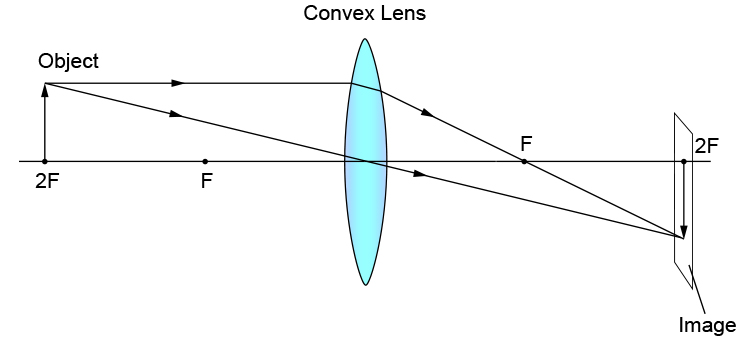# Convex lens – object at 2F

This means the object is two principal focal lengths from the convex lens.

A ray diagram can be constructed using the two standard rules

1. From the object, pass a parallel line through the principal focal point
2. From the object, pass a ray line through the centre of the convex lens.We can see that when the object is two principal focal lengths from the convex lens, the image can appear on a screen exactly at two principal focal lengths on the other side of the lens. The image will be inverted but can be captured on a screen.